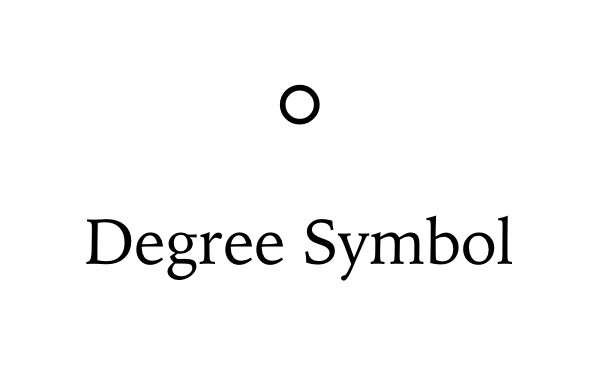# Degree Symbol

Degree symbol is °. Sometimes students or those who deal with mathematics, physics or various kinds of calculations may need to type a degree sign, but we do not have one directly on our keyboard. Degree symbol can be used in case if we're dealing with angles, or when we need to operate with temperature and use Celsius degree. It is also a common coordinate degree sign. Actually, there are a few ways to make a degree symbol. You can either copy paste, or use the degree code in your document. See some examples below:

» Degree symbol on Windows » Degree symbol on Mac
» Degree symbol examples
° &#176;

html degree decimal

° &#xb0;

html degree hex

° &deg;

html degree named

## Degree Symbol on Microsoft Windows PC

Press and hold the ALT key and type 0176 on the numeric keypad of your keyboard. Make sure the NumLock is on and type 0176 with the leading zero. If there is no numeric keypad, press and hold the Fn before typing the 0176 numbers of degree symbol.

## Degree Symbol on Mac OS

Press and hold the SHIFTOPTION8 keys on your keyboard.

### Degree Symbol in LaTeX

Degree symbol can be typed in LaTeX with using packages.

textcomp
\usepackage{textcomp}
\begin{document}
Degree symbol: 90\si{\textdegree}
\end{document}

gensymb
\usepackage{gensymb}
\begin{document}
Degree symbol: 90\si{\degree}
\end{document}

siunitx
\usepackage{siunitx}
\begin{document}
Degree symbol: 90\si{\degree}
\end{document}

circ
^{\circ}


### Celsius Degree Symbol

&#8451;

celsius degree decimal

&#x2103;

celsius degree hex

\u2103

celsius degree source code

### Fahrenheit Degree Symbol

&#8457;

fahrenheit degree decimal

&#x2109;

fahrenheit degree hex

\u2109

fahrenheit degree source code

### Masculine Ordinal Indicator Symbol

º &#186;

degree decimal

º &#xba;

degree hex

º \u00BA

degree source code

### Ring Above Symbol

˚ &#730;

degree decimal

˚ &#x2da;

degree hex

˚ \u02DA

degree source code

### Ring Below Symbol

̥ &#805;

degree decimal

̥ &#x325;

degree hex

̥ \u0325

degree source code

### Superscript Zero Symbol

&#8304;

degree decimal

&#x2070;

degree hex

\u2070

degree source code

### Ring Operator Symbol

&#8728;

degree decimal

&#x2218;

degree hex

\u2218

degree source code

#### Degree Symbol Examples

Degree symbol in geometry.

• Right angle is 90°. (90 degrees)
• Straight angle is 180°. (180 degrees)
• Acute angle is less than 90°. (<90 degrees)
• Obtuse angle is greater than 90° and less than 90°. (>90 degrees and <180 degrees)
• Reflex angle is greater than 180°. (>180 degrees)

Degree symbol in geographic coordinate system.

• Latitude 32.344790 is equal to 32° 20' 41.244'' N. (32 degrees North)
• Longitude -64.692307 is equal to 64°° 41' 32.3052'' W. (64 degrees West)

Degree symbol in temperature measurements.

• 2°C : 2 degrees Celsius
• 20°C : 20 degrees Celsius
• 23°C : 23 degrees Celsius
• 10℃ : 10 degrees Celsius
• 15℃ : 15 degrees Celsius
• -21℃ : -21 degrees Celsius